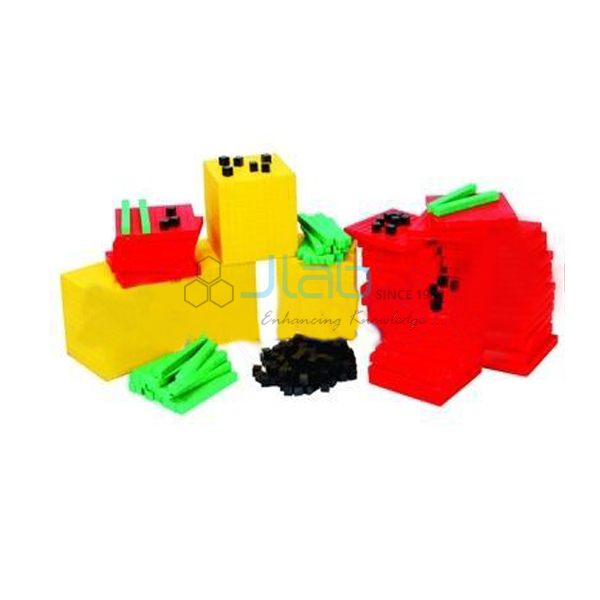Base Ten BlockProduct Code : JL-MLI-1737

Base Ten Block

Students learn math lessons faster when they can see a visual demonstration of the lesson using base ten Math manipulative.

Based on the centimetre cube, Base Ten Blocks are valuable and versatile manipulative for any mathematics lessons.

Base ten block help students understand place value and measurement concepts related to surface and volume.

This Base Ten Beginner Set includes 100 unit cubes, 20 ten rods, 10 hundred flats, and 1 thousand cube.